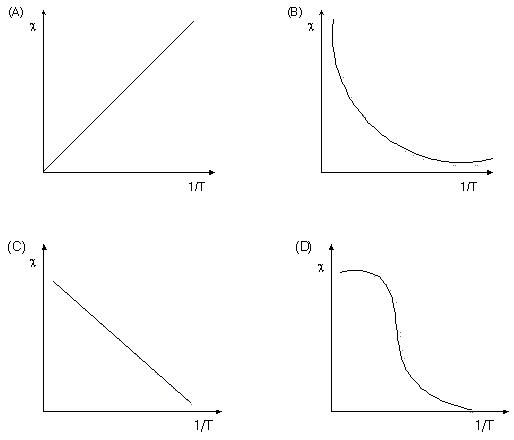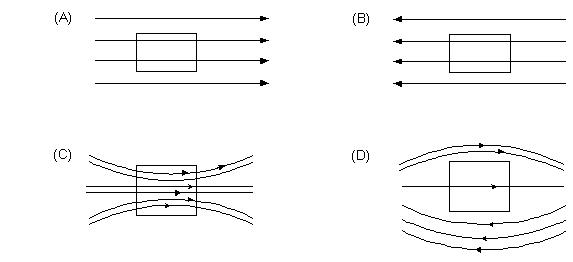×#### Thank you for registering.

One of our academic counsellors will contact you within 1 working day.

Click to Chat

1800-1023-196

+91-120-4616500

CART 0

• 0

MY CART (5)

Use Coupon: CART20 and get 20% off on all online Study Material

ITEM
DETAILS
MRP
DISCOUNT
FINAL PRICE
Total Price: Rs.

There are no items in this cart.
Continue Shopping• Complete Physics Course - Class 11
• OFFERED PRICE: Rs. 2,968
• View Details

```Assignment and Solutions

1.       The relation between μ and H for a specimen of iron is as follows-

μ = [0.4/H + 12 × 10–4] Henry / meter

The value of H which produces flux density of 1 Tesla will be-

(A) 250 A/m                                                   (B) 500 A/m   (C) 750 A/m   (D) 103 A/m

Solution:(B) B = μH

or   B = [0.4 / H + 12 × 10–4] H

or   1 = 0.4 + 12×10–4 H

H = 500 A/m

2.       The correct curve between X and 1/T  for paramagnetic materials is-Solution:        (A) X ∝ 1/T

3.       The SI unit of magnetic flux is-

(A)       Weber            (B)       Maxwel          (C)       Tesla              (D)       Gauss

Solution:        (A) Φ = BA = Weber m2/m2 = Weber

4.       The intensity of magnetic field due to an isolated pole of strength mp at a point distant r from it will be-

(A) mp / r2                      (B)       mpr2                (C)       r2 / mp              (D) mp / r

Solution:        (A) The magnetic intensity due to an isolated pole of strength mp at a distance r = mp / r2

5.       A uniform magnetic field is directed from left towards right in the plane of paper. When a piece of soft iron is placed parallel to the field. The magnetic lines of force passing through it will be-Solution:        (C) The magnetic lines of force in a ferrmagnetic material are crowded together.

6.       A bar magnet of magnetic moment M is cut into two equal parts. The magnetic moment of either of the parts will be-

(A) M/2                         (B)       2M                   (C)       2M                   (D)       l ∝ H2

Solution:        (A) M = ml M' = m l/2 = M/2

7.       A loop of area 0.5 m2 is placed in a magnetic field of strength 2 Tesla in direction making an angle of 60o with the field. The magnetic flux linked with the loop will be-

(A) 1/2 Weber             (B) √3/2 Weber           (C)       2 Weber         (D)       √3 Weber

Solution:        (A) Φ = BA cos θ, Φ = 2 × 1/2 cos 60o = 1/2

8.       The force experienced by a pole of strength 100 A-m at a distance of 0.2m from a short magnet of length 5 cm and pole strength of 200A-m on its axial line will be

(A)       2.5×10–2 N                                         (B)       2.5×10–3N

(C)       5.0×10–2N                                          (D)       5.0×10–3N.

Solution:        (A) F = mB = μ0 / 4π 2m'l / x3 m

= 10–7 × 2 × 200 × 0.05 × 100 / 8 × 10–3

= 2.5×10–2 N

9.       The magnetic susceptibility of a paramagnetic substance is 3×10–4. It is placed in a magnetising field of 4×104 amp/m. The intensity of magnetisation will be-

(A)       3 × 108 A/M                                        (B)       12 × 108 A/M

(C)       12 A/M                                                (D)       24 A/M

Solution:        (C) I = XH = 3×10–4×4×103 = 12 A/m

10.       Volt-second is the unit of-

(A)       B                     (B)       Φ                      (C)       I                       (D)       x

Solution:        (B) e = dΦ / dt or Φ = et = volt – sec

11.          The volume susceptibility of a magnetic material is 30×10–4. Its relative permeability will be-

(A)       31 × 10–4        (B)       1.003              (C)       1.0003            (D)       29 × 10–4

Solution:        (B) μr = μ/μ0 = 1 + X = 1 + 30 × 10–4 = 1.003

12.       A current of 2 ampere is passed in a coil of radius 0.5 m and number of turns 20. The magnetic moment of the coil is-

(A)       0.314 A–m2                                       (B)       3.14 A–m2

(C)       314 A–m2                                                        (D)       31.4 A–m2

Solution:        (D) M = i R2N = 2×3.14×0.25×20 = 31.4 A–m2

13.       The value of magnetic susceptibility for super-conductors is-

(A)       zero                (B)       infinity            (C)       +1                    (D)       –1

Solution:        (D) For superconductor

μr = 1 + X = 0              X = –1

14.       A current of 1 ampere is flowing in a coil of 10 turns and with radius 10 cm. Its magnetic moment will be-

(A)       0.314 A-m2                                        (B)       3140 A-m2

(C)       100 A-m2                                           (D)       m0 A-m2

Solution:        (A) M = iA = πR2 Ni

= 3.14×0.01×10×1=0.314 Am2

15.       The magnetic moment of a magnet of mass 75 gm is 9×10–7 A-m2. If the density of the material of magnet is 7.5×103 kg/m3 then intensity of magnetisation will be-

(A)       0.9 A/m          (B)       0.09 A/m        (C)       9 A/m              (D)       90 A/m

Solution:        (B) l = M/V = Md / m = 9 × 10–7 × 7.5 × 103/75 × 10–3 = 0.09 A/m
```### Course Features

• 101 Video Lectures
• Revision Notes
• Previous Year Papers
• Mind Map
• Study Planner
• NCERT Solutions
• Discussion Forum
• Test paper with Video Solution